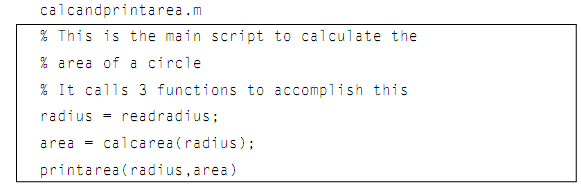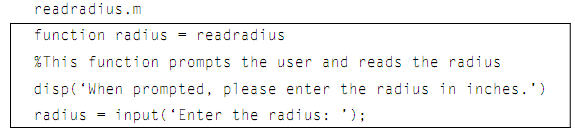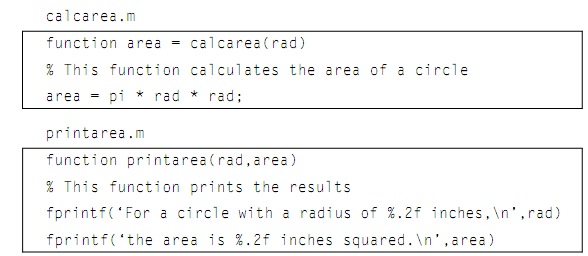## Example of modular program, MATLAB in Engineering

Assignment Help:

Example of modular program:

In a modular program, there would be one main script which calls three separate functions to complete these tasks:

• A function to prompt an user and read in the radius
• A function to compute the area of a circle
• A function to show the results

As both the scripts and functions are stored in M-files, there would be four individual M-files together for this program; one M-file script and three M-file functions, as shown below:#### Function numden, function numden: The function numden will return indi...

function numden: The function numden will return individually the numerator & denominator of a symbolic expression: >> sym(1/3 + 1/2) ans = 5/6 >> [n, d] =

#### Help command, Help command: The help command is used with the script r...

Help command: The help command is used with the script rectarea, the function readlenwid, and the major function printrectarea. To see the first comment in the subfunction, as

#### Program to examine exponential function, Write a program to examine exponen...

Write a program to examine exponential function: We will write a program to examine the value of e and the exponential function. It will be a menu-driven. The menu options wil

#### Illustration of finding a sting, Illustration of finding a sting: Le...

Illustration of finding a sting: Let's enlarge this, and write a script which creates a vector of strings which are phrases. The outcome is not suppressed so that the string

#### Algorithm for subfunction, Algorithm for subfunction: The algorithm fo...

Algorithm for subfunction: The algorithm for subfunction askforn is as shown:  Prompt the user for the positive integer n.  Loop to print an error message and reprom

#### Examine exponential function - algorithm, Examine exponential function: ...

Examine exponential function: The algorithm for the main script program is shown below:  Call a function eoption to show the menu and return the user's choice.  Loop

#### Finding sums by for loop, Finding sums and products: A very general ap...

Finding sums and products: A very general application of a for loop is to compute sums and products. For illustration, rather than of just printing the integers 1 through 5, w

#### Square matrices, Square Matrices: If a matrix has similar number of ro...

Square Matrices: If a matrix has similar number of rows and columns, for illustration, if m == n, the matrix is square matrix. The definitions which follow in this part apply

#### Technique to creating this structure, Technique to creating this structure:...

Technique to creating this structure: An alternative technique of creating this structure, that is not as efficient, includes using the dot operator to refer to fields in the

#### Indexing into vectors of structures, Indexing into Vectors of structures: ...

Indexing into Vectors of structures: Frequently, when the data structure is a vector of structures, it is essential to iterate through the vector in order by various fields. F